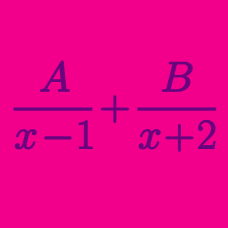Algebra

# Partial Fractions: Level 3 Challenges

$\frac{1}{2!} + \frac{2}{3!} + \frac{3}{4!} + \frac{4}{5!} + \cdots = \ ?$

$1 + \frac {1}{\color{#3D99F6}2} + \frac {1}{\color{#3D99F6}6} + \frac {1}{\color{#3D99F6}{12}} + \frac {1}{\color{#3D99F6}{20}} + \ldots = \ \color{teal}?$

$\large \displaystyle \sum_{n = 1}^{\infty} \dfrac {1}{n^2 + 3n + 2} = \ ?$

$\frac{1}{(x-1)(x-2)} + \frac{1}{(x-2)(x-3)} + \frac{1}{(x-3)(x-4)} = \frac{1}{6}$

What is the sum of all real values of $x$ that satisfy the above equation?

$\LARGE \sqrt{ \sqrt{32}} \sqrt{ \sqrt{32}} \sqrt{ \sqrt{32}} \cdots \sqrt{ \sqrt{32}} = \ ?$

×

Problem Loading...

Note Loading...

Set Loading...